Download Presentation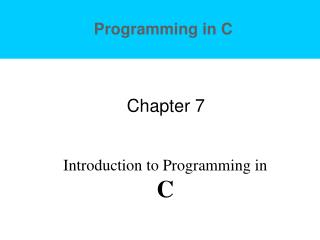Chapter 7

# Chapter 7 - PowerPoint PPT Presentation

Chapter 7. Introduction to Programming in C. Program 6 - Solution. int main() { int i, n, d; /* Counter, number of days in month, number in week */ printf("Enter the number of days in the month:"); scanf("%d" , &n);I am the owner, or an agent authorized to act on behalf of the owner, of the copyrighted work described.
Download Presentation## Chapter 7

An Image/Link below is provided (as is) to download presentation

Download Policy: Content on the Website is provided to you AS IS for your information and personal use and may not be sold / licensed / shared on other websites without getting consent from its author.While downloading, if for some reason you are not able to download a presentation, the publisher may have deleted the file from their server.

- - - - - - - - - - - - - - - - - - - - - - - - - - E N D - - - - - - - - - - - - - - - - - - - - - - - - - -
Presentation Transcript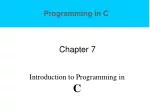### Chapter 7

Introduction to Programming in C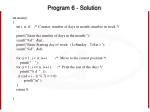Program 6 - Solution

int main()

{

int i, n, d; /* Counter, number of days in month, number in week */

printf("Enter the number of days in the month:");

scanf("%d" , &n);

printf("Enter Starting day of week: (1=Sunday , 7=Sat.):");

scanf("%d" , &d);

for (i = 1; i < d; i++) /* Move to the correct position */

printf(" ");

for (i = 1; i <= n; i++) { /* Print the rest of the days */

printf("% d " , i);

if (((d + i - 1) % 7) = = 0)

printf("\n" );

}

return 0;

}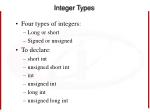Integer Types
• Four types of integers:
• Long or short
• Signed or unsigned
• To declare:
• short int
• unsigned short int
• int
• unsigned int
• long int
• unsigned long int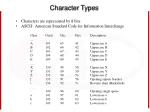Character Types
• Characters are represented by 8 bits
• ASCII: American Standard Code for Information Interchange

Char Octal Dec Hex Description

....

A 101 65 41 Uppercase A

B 102 66 42 Uppercase B

C 103 67 43 Uppercase C

D 104 68 44 Uppercase D

E 105 69 45 Uppercase E

.....

X 130 88 58 Uppercase X

Y 131 89 59 Uppercase Y

Z 132 90 5a Uppercase Z

[ 133 91 5b Opening square bracket

\ 134 92 5c Reverse slant (Backslash)

.....

` 140 96 60 Opening single quote

a 141 97 61 Lowercase a

b 142 98 62 Lowercase b

c 143 99 63 Lowercase c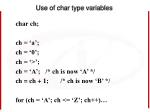Use of char type variables

char ch;

ch = ‘a’;

ch = ‘0’;

ch = ‘>’;

ch = ‘A’; /* ch is now ‘A’ */

ch = ch + 1; /* ch is now ‘B’ */

for (ch = ‘A’; ch <= ‘Z’; ch++)…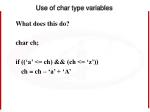Use of char type variables

What does this do?

char ch;

if ((‘a’ <= ch) && (ch <= ‘z’))

ch = ch – ‘a’ + ‘A’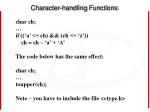Character-handling Functions

char ch;

if ((‘a’ <= ch) && (ch <= ‘z’))

ch = ch – ‘a’ + ‘A’

The code below has the same effect:

char ch;

toupper(ch);

Note – you have to include the file <ctype.h>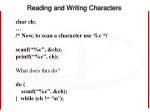Reading and Writing Characters

char ch;

/* Now, to scan a character use %c */

scanf(“%c”, &ch);

printf(“%c”, ch);

What does this do?

do {

scanf(“%c”, &ch);

} while (ch != ‘\n’);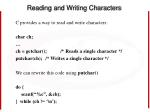Reading and Writing Characters

C provides a way to read and write characters:

char ch;

ch = getchar(); /* Reads a single character */

putchar(ch); /* Writes a single character */

We can rewrite this code using putchar()

do {

scanf(“%c”, &ch);

} while (ch != ‘\n’);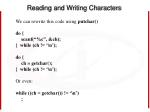Reading and Writing Characters

We can rewrite this code using putchar()

do {

scanf(“%c”, &ch);

} while (ch != ‘\n’);

do {

ch = getchar();

} while (ch != ‘\n’);

Or even:

while ((ch = getchar()) != ‘\n’)

;Reading and Writing Characters

We can rewrite this:

while ((ch = getchar()) != ‘\n’)

;

In an even simpler form:

while (getchar() != ‘\n’)

;

Also, can test for another character. What does this do?

while (getchar() != ‘Z’)

;Reading and Writing Characters

/* Determines the length of a message */

#include <stdio.h>

main()

{

char ch;

int len = 0;

printf("Enter a message: ");

ch = getchar();

while (ch != '\n') {

len++;

ch = getchar();

}

printf("Your message was %d character(s) long.\n", len);

return 0;

}Reading and Writing Characters

/* Determines the length of a message */

#include <stdio.h>

main()

{

int len = 0;

printf("Enter a message: ");

while (getchar() != '\n')

len++;

printf("Your message was %d character(s) long.\n", len);

return 0;

}Reading and Writing Characters

/* Determines the length of a message */

#include <stdio.h>

main()

{

int len = 0;

printf("Enter a message: ");

while (getchar() != '\n')

len++;

printf("Your message was %d character(s) long.\n", len);

return 0;

}sizeof Operator

The sizeof operator allows the program to determine how many bytes of memory are required to store values of a particular data type.

Format: sizeof (type)

Example:

printf("the int is %d\n", sizeof(int));

sizeof (char) is always 1

sizeof(int) is 4

sizeof(double) is 8Type Conversions

Type conversion: convert a unit of data from one type to another

Automatic in some cases:

• Add an int to a long int: compiler converts the int to a long int
• Add an int to a float: compiler converts the int to a float

“Implicit conversions” – compiler handles automatically

“Explicit conversions” – use the cast operatorImplicit Conversions
• When the operands in an arithmetic or logical expression don’t have the same type
• When the type of the expression on the right side of an assignment doesn’t match that on the left side
• When the type of an argument in a function call doesn’t match the type of the corresponding parameter
• When the type of the expression in the return statement doesn’t match the function’s return typeExamples – Arithmetic Conversions

char c; long int l;

short int s; unsigned long int ul;

int i; float f;

unsigned int u; double d;

long double ld;

i = i + c; /* c is converted to int */

i = i + s; /* s is converted to int */

u = u + i; /* i is converted to unsigned int */

l = l + u; /* u is converted to long int */

i = i + c; /* c is converted to int */

ul = ul + l; /* l is converted to unsigned long int */

f = f + ul; /* ul is converted to float */

d = d + f; /* f is converted to double */

ld = ld + d; /* d is converted to long double */Examples – Conversions During Assignment

char c;

int i;

float f;

double d;

i = c; /* c is converted to int */

f = i; /* i is converted to float */

d = f; /* f is converted to double */Some BAD Ideas – DON’T DO THIS

char c;

int i;

float f;

double d;

i = 57.33; /* NO! */

i = 1.0e20; /* NO, NO!! */

f = 1.0e100; /* Nope. */

c = 10000; /* Won’t work */Type Casting

C allows us to “cast” an expression.

(type) expression;

For example – assume you have a float

f = 100.00;

And you happen to need to use it as an integer for assignment to an integer variable:

i = (int) f; /* Converts f to an integer */